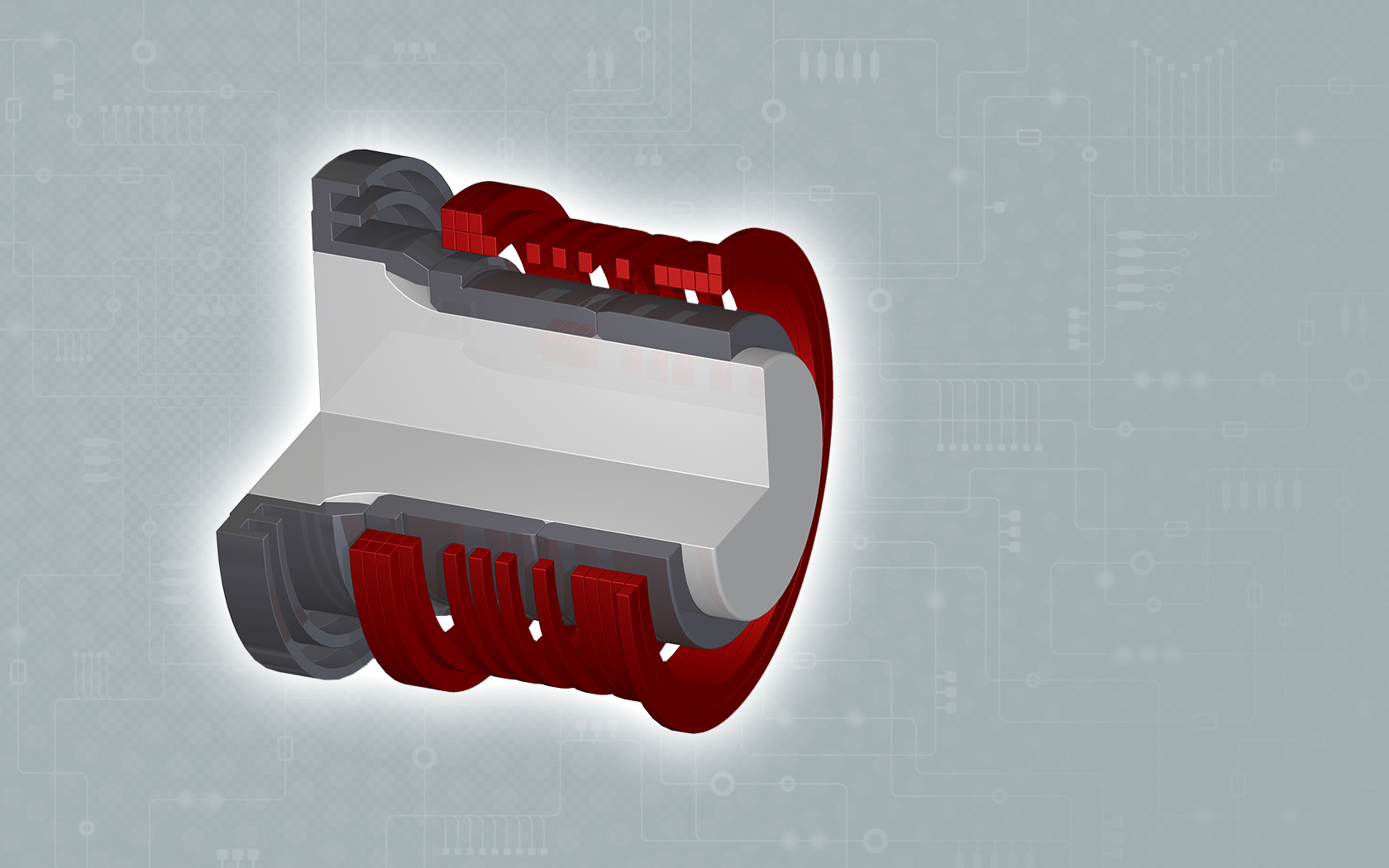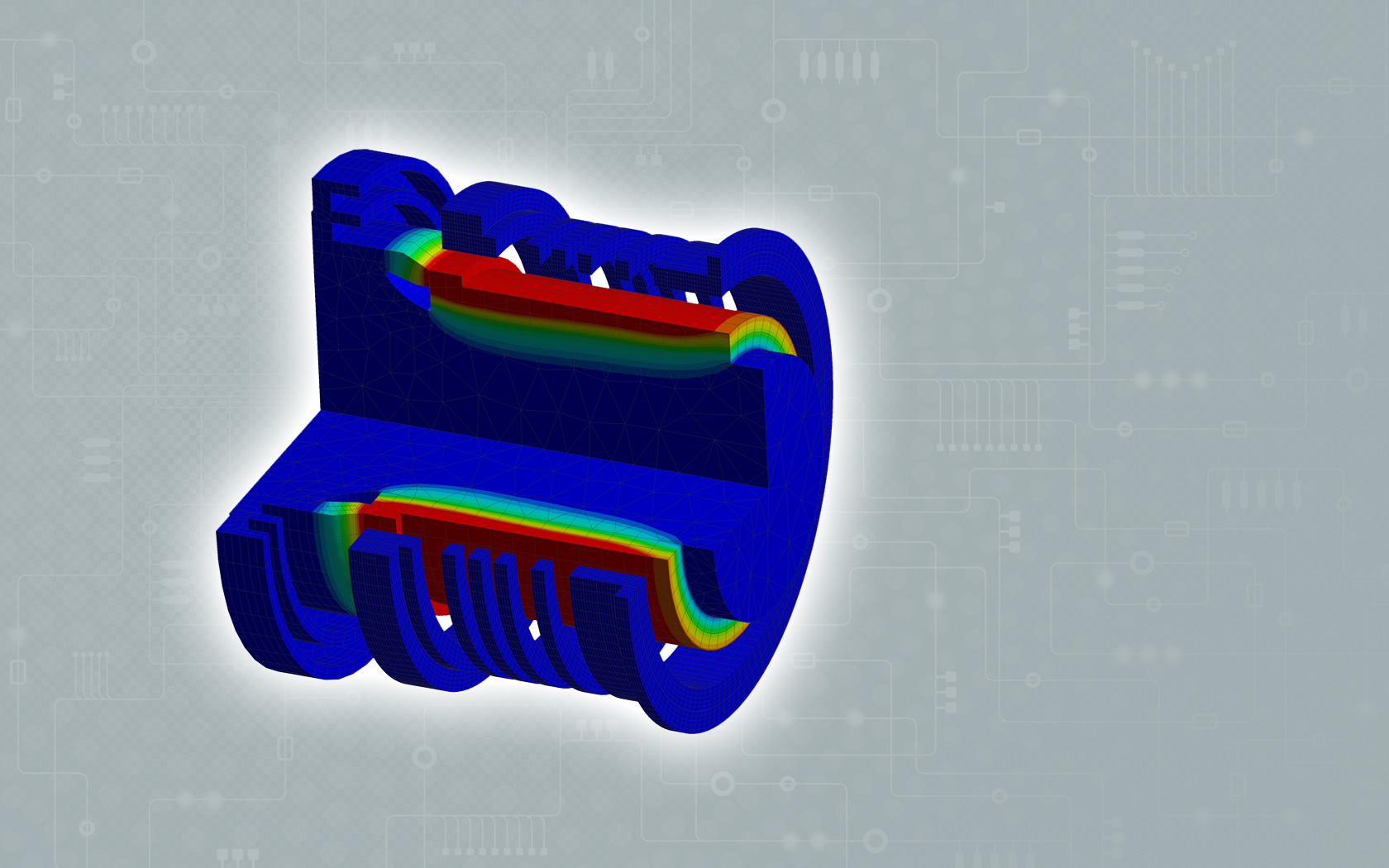## Power calculator | induction heating of steel

Calculation examples for induction heating of steel / iron with tempreratures up to 300 °C

Example for a cylindrical shrink fit      Example for generator power
Work piece or part
outside diameter

mm

Work piece or part
innerdiameter

mm

Width to be heated
mm
Mass to be heated
kg
Generator power
kW
Temperature difference
K
Shrink fit dimension
mm
Heating time
sec.
Results:   Results:
Heated mass
kg
Needed power *
kW
Needed temperature
difference

K

Heating time*
sec.

Costs per kWh
Cent
Costs per kWh
Cent
Results:   Results:
Energy costs per part
Cent
Energy costs per part
Cent

*Losses of heat radiation and conductivity are not included.

## Estmation of needed power

In general the electrical power of the generator can be estimated as follows considering a certain mass, a preset time and a preset temperature of the work piece:

P • t   =   m • c • ΔT

P         Generator power [kW]
t           Heating time [s]
m         Mass Work piece [kg]
c          Specific heat capacity [kW s / kg K]
ΔT       Temperature difference [K]  (End temperature minus Starting temperature)